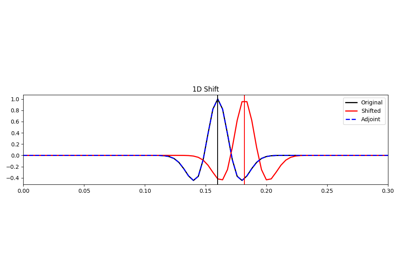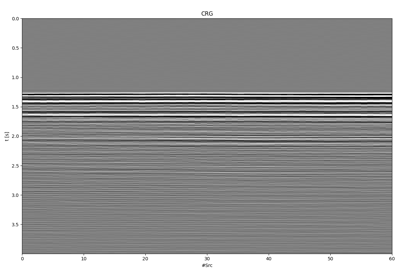# pylops.signalprocessing.Shift¶

pylops.signalprocessing.Shift(dims, shift, axis=-1, nfft=None, sampling=1.0, real=False, engine='numpy', dtype='complex128', name='S', **kwargs_fftw)[source]

Shift operator

Apply fractional shift in the frequency domain along an axis of a multi-dimensional array of size dims.

Parameters
dimstuple

Number of samples for each dimension

shift

Fractional shift to apply in the same unit as sampling. For multi-dimensional inputs, this can be a scalar to apply to every trace along the chosen axis or an array of shifts to be applied to each trace.

axisint, optional

New in version 2.0.0.

Axis along which shift is applied

nfftint, optional

Number of samples in Fourier Transform (same as input if nfft=None)

samplingfloat, optional

Sampling step $$\Delta t$$.

realbool, optional

Model to which fft is applied has real numbers (True) or not (False). Used to enforce that the output of adjoint of a real model is real.

enginestr, optional

Engine used for fft computation (numpy, scipy, or fftw). Choose numpy when working with CuPy arrays.

dtypestr, optional

Type of elements in input array.

namestr, optional

New in version 2.0.0.

Name of operator (to be used by pylops.utils.describe.describe)

**kwargs_fftw

Arbitrary keyword arguments for pyfftw.FTTW

Raises
ValueError

If dims is provided and axis is bigger than len(dims)

NotImplementedError

If engine is neither numpy, scipy, nor fftw

Notes

The Shift operator applies the forward Fourier transform, an element-wise complex scaling, and inverse fourier transform

$\mathbf{y}= \mathbf{F}^{-1} \mathbf{S} \mathbf{F} \mathbf{x}$

Here $$\mathbf{S}$$ is a diagonal operator that scales the Fourier transformed input by $$e^{-j2\pi f t_S}$$, where $$t_S$$ is the chosen shift.

Attributes
shapetuple

Operator shape

explicitbool

Operator contains a matrix that can be solved explicitly (True) or not (False)

## Examples using pylops.signalprocessing.Shift¶Shift

Shift18. Deblending

18. Deblending